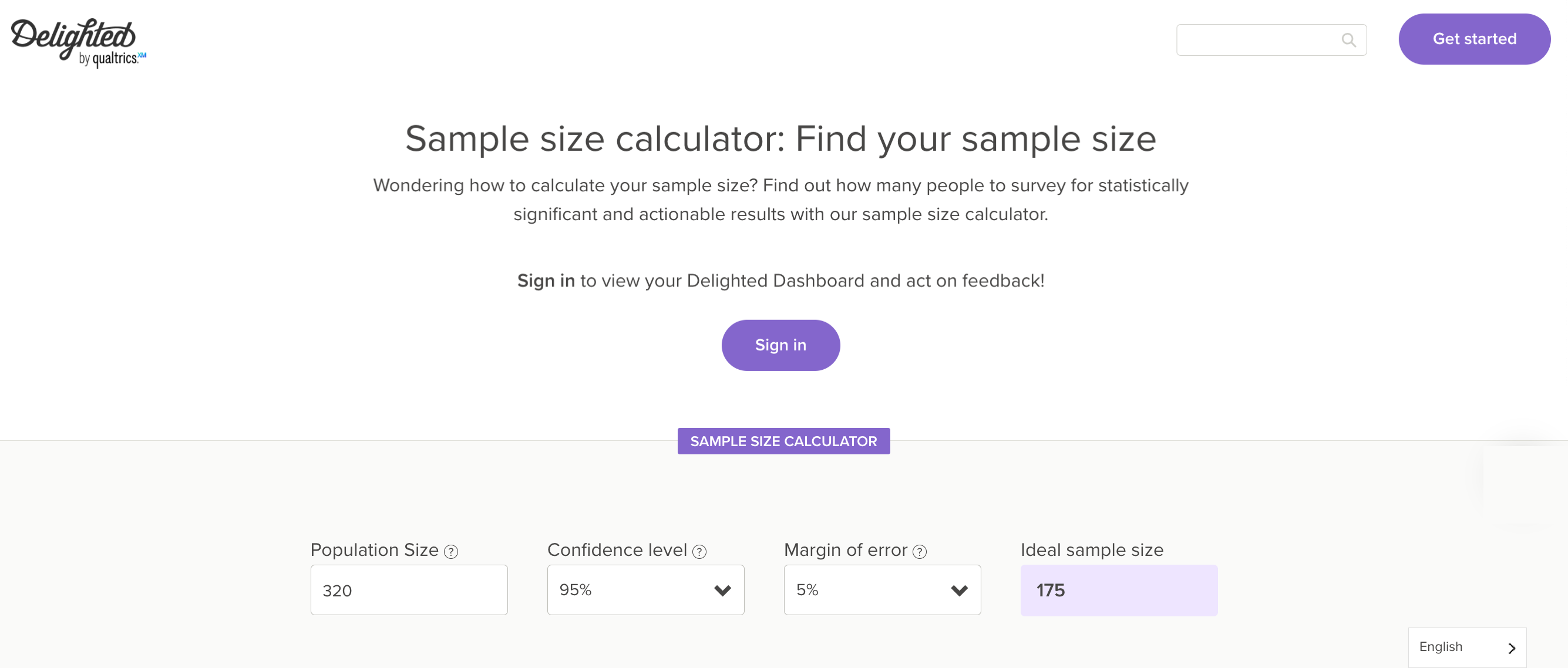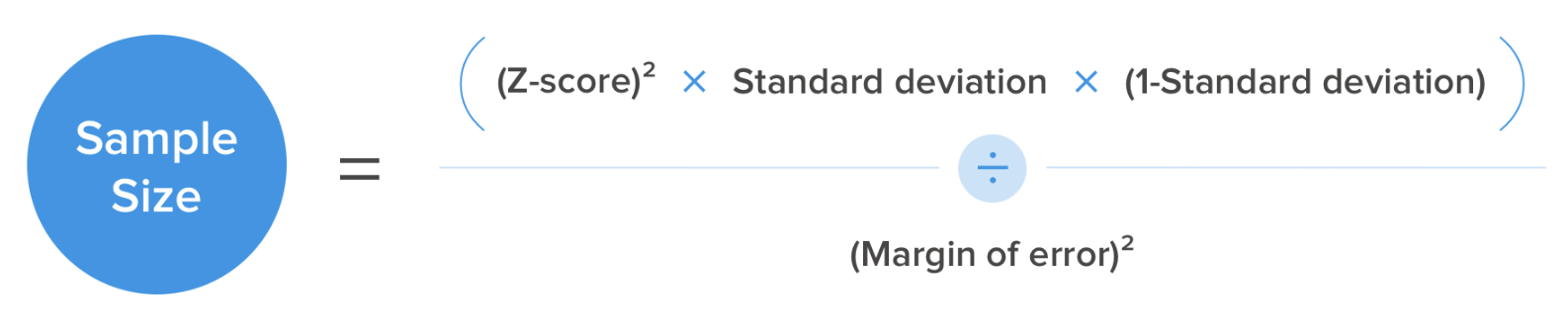# Sample Size Calculation

At Delighted, we like to make things simple — so we created a Sample Size calculator that you can use to estimate the number of people you’ll need to survey to collect accurate results. ("Sample size" is the number of people you should survey to gather results within a margin of error.)The formula

Formulas are applied to determine the appropriate sample size for a survey. A commonly applied formula is:Breaking down the formula

There are a number of factors to consider, including:

• The population — What is the size of the overall population the you are surveying?
• The margin of error — What is the margin of error (ex. 5%) that you are willing to accept?
• The confidence level — What is the confidence level you need (ex. 95%) to verify  that your results are accurate?

n = (Z^2 * p * (1-p)) / E^2

Where:

• n = sample size
• Z = z-score for the desired confidence level (1.96 for a 95% confidence level)
• p = population proportion (estimate of the percentage of respondents with a particular characteristic)
• E = margin of error (5% in this case)

Glance at the example entered into the image of Delighted's sample size calculator above. In this, we imagined that  320 people attended a Museum Fundraiser.  We wanted to know how satisfied they were with the event. And we wanted a 95% confidence level with a margin of error of 5%.

Plugging that information into the formula, the sample size would be 175.